# NCERT Solutions class 7 Maths Chapter-10 Exercise 10.3

NCERT Solutions Class-7 Maths chapter-10 line and angle Exercise-10.3 is prepared by academic team of pw all the questions of NCERT text book are solved step by step with proper and detail solutions explaining each and every questions . For More and additional questions of CBSE class 7 maths you can go to class 7 maths sections. NCERT class 7 Maths Solutions is the best way to enhanced your mathematics skill. And pw practice worksheet & question bank will help you a lot .

## NCERT Solutions class 7 Maths Chapter-10 line and angle

### Solutions of Chapter line and angle Exercise-10.3

Question 1:
Construct DEF such that DE = 5 cm, DF = 3 cm and m∠EDF = 90°.
The rough sketch of the required ?DEF is as follows.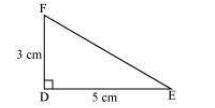The steps of construction are as follows.
(i)Draw a line segment DE of length 5 cm.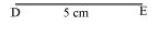(ii) At point D, draw a ray DX making an angle of 90° with DE.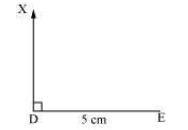(iii) Taking D as centre, draw an arc of 3 cm radius. It will intersect DX at point F.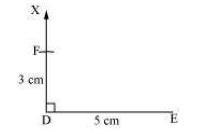(iv) Join F to E. ?DEF is the required triangle.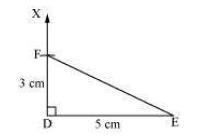Question 2:
Construct an isosceles triangle in which the lengths of each of its equal sides is 6.5 cm and the angle between them is 110°.
An isosceles triangle PQR has to be constructed with PQ = QR = 6.5 cm. A rough sketch of the required triangle can be drawn as follows.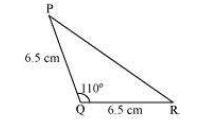The steps of construction are as follows.
(i) Draw the line segment QR of length 6.5 cm.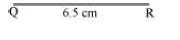(ii) At point Q, draw a ray QX making an angle 110° with QR.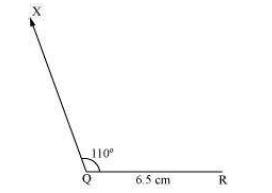(iii) Taking Q as centre, draw an arc of 6.5 cm radius. It intersects QX at point P.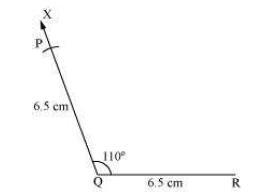(iv) Join P to R to obtain the required triangle PQR.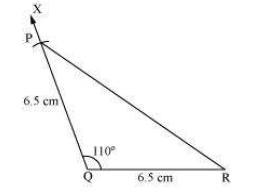Question 3:
Construct ?ABC with BC = 7.5 cm, AC = 5 cm and m∠C = 60°.
A rough sketch of the required triangle is as follows.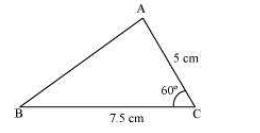The steps of construction are as follows.
(i) Draw a line segment BC of length 7.5 cm.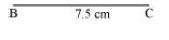(ii) At point C, draw a ray CX making 60º with BC.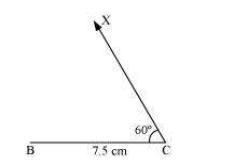(iii) Taking C as centre, draw an arc of 5 cm radius. It intersects CX at point A.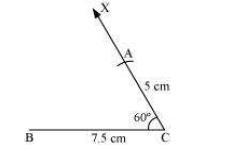(iv) Join A to B to obtain triangle ABC.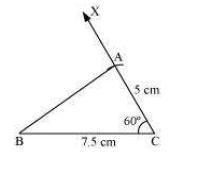NCERT CLASS 7 MATHEMATICS SOLUTIONS